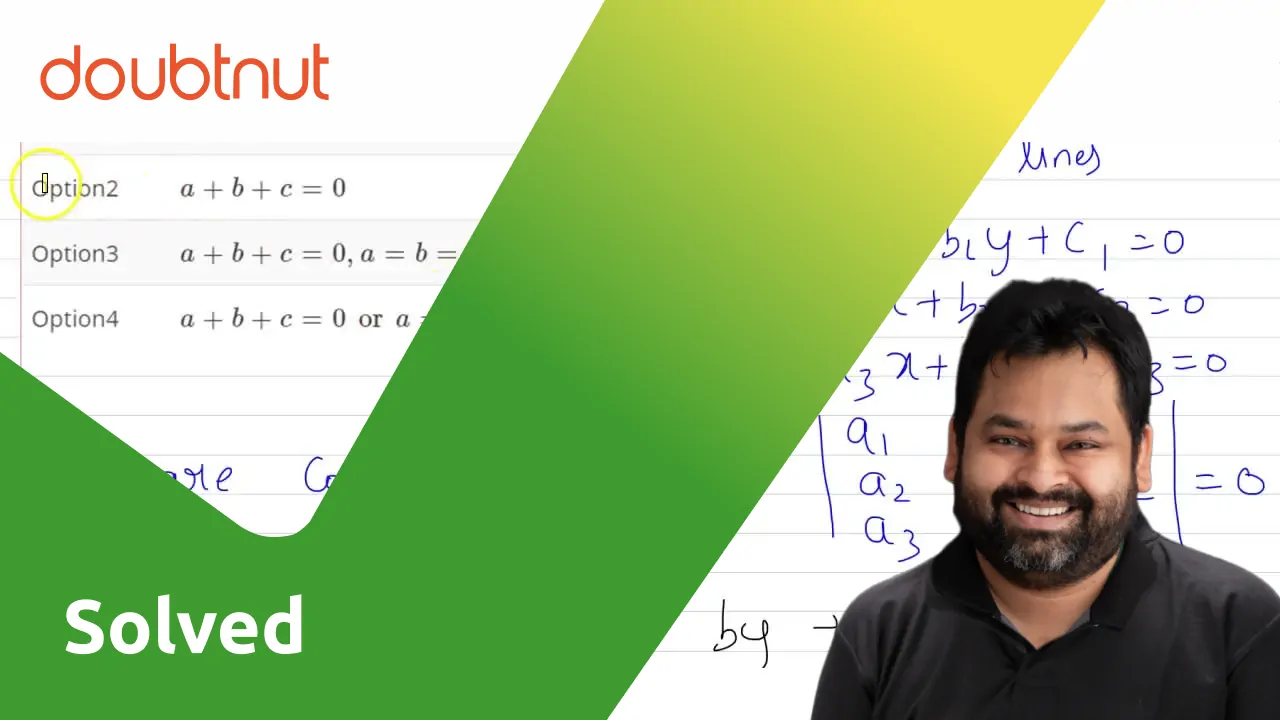Doubtnut App - Get 50 Lakh+ video solutions better than GoogleInstall Now

# The condition that the three different lines ax+by+c=0,bx+cy+a=0,cx+ay+b=0 to be concurrent is Solution in Telugu

A

a=b=c

B

a+b+c=0

C

a+b+c=0,a=b=c

D

a+b+c=0ora=b=c

Text Solution
Verified by Experts

Video Solution